Related Articles
Class 8 RD Sharma Solutions – Chapter 18 Practical Geometry – Exercise 18.4
• Last Updated : 05 May, 2021

### Question 1: Construct a quadrilateral ABCD in which AB = 6 cm, BC = 4 cm, CD = 4 cm, ∠B = 95° and ∠C = 90°?

Solution:

We have, AB = 6 cm, BC = 4 cm, CD = 4 cm, ∠B = 95° and ∠C = 90°.

Step 1: Draw a line segment BC = 4cm

Step 2: Construct an angle of 95° at B.

Step 3: Taking B as the centre, draw an arc radius of 6 cm. Mark this point as A.

Step 4: Construct an angle of 90° at C.

Step 5: Taking C as the centre, draw an arc radius of 4 cm. Mark this point as D.

Step 6: Now join the line segments BA, CD, and AD to form a quadrilateral.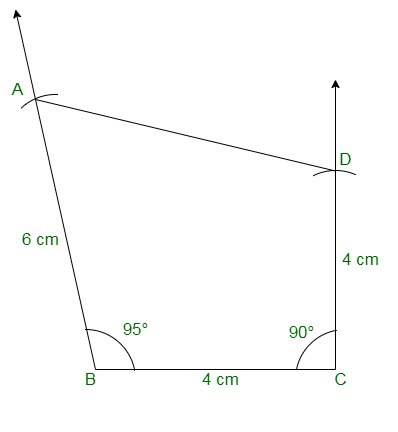### Question 2: Construct a quadrilateral ABCD where AB = 4.2cm, BC = 3.6 cm, CD = 4.8 cm, ∠B = 30° and ∠C = 150°?

Solution:

The given details are AB = 4.2cm, BC = 3.6 cm, CD = 4.8 cm, ∠B = 30° and ∠C = 150°.

Step 1: Draw a line segment BC = 3.6cm

Step 2: Construct an angle of 30° at B.

Step 3: Taking B as the centre, draw an arc radius of 4.2 cm. Mark this point as A.

Step 4: Construct an angle of 150° at C.

Step 5: Taking C as the centre, draw an arc radius of 4.8 cm. Mark this point as D.

Step 6: Now join BA, CD, and AD to form a quadrilateral.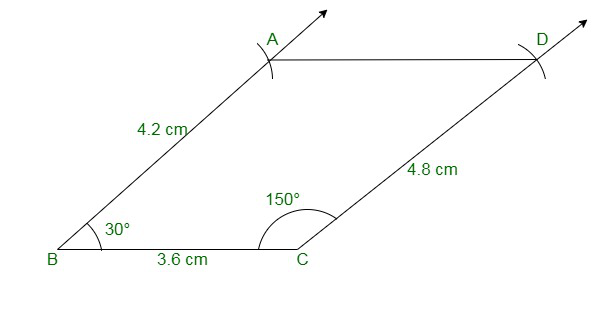### Question 3: Construct a quadrilateral PQRS in which PQ = 3.5 cm, QR = 2.5 cm, RS = 4.1 cm, ∠Q = 75° and ∠R = 120°?

Solution:

The given details are PQ = 3.5 cm, QR = 2.5 cm, RS = 4.1 cm, ∠Q = 75° and ∠R = 120°.

Step 1: Draw a line segment QR = 2.5cm

Step 2: Construct an angle of 75° at Q.

Step 3: Taking Q as the centre, draw an arc radius of 3.5 cm. Mark this point as P.

Step 4: Construct an angle of 120° at R.

Step 5: Taking R as the centre, draw an arc radius of 4.1 cm. Mark this point as S.

Step 6: Now join QP, RS, and PS to form a quadrilateral.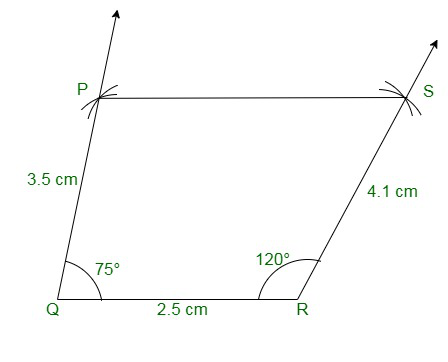### Question 4: Construct a quadrilateral ABCD given BC = 6.6 cm, CD = 4.4 cm, AD = 5.6 cm ∠D = 100° and ∠C = 95?

Solution:

We have, BC = 6.6 cm, CD = 4.4 cm, AD = 5.6 cm ∠D = 100° and ∠C = 95

Step 1: Draw a line segment DC = 4.4cm

Step 2: Construct an angle of 100° at D.

Step 3: Taking D as the centre, draw an arc radius of 5.6 cm. Mark this point as A.

Step 4: Construct an angle of 95° at C.

Step 5: Taking C as the centre, draw an arc radius of 6.6 cm. Mark this point as B.

Step 6: Now join DA, CB, and AB to form a quadrilateral.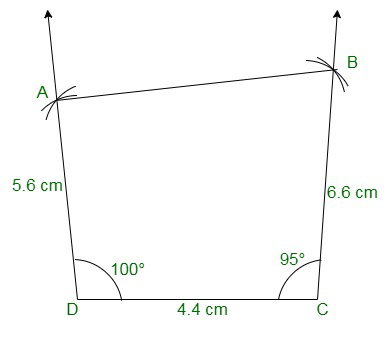### Question 5: Construct a quadrilateral ABCD in which AD = 3.5 cm, AB = 4.4 cm, BC = 4.7 cm, ∠A = 125° and ∠B = 120°?

Solution:

The given details are AD = 3.5 cm, AB = 4.4 cm, BC = 4.7 cm, ∠A = 125° and ∠B = 120°.

Step 1: Draw a line AB = 4.4cm

Step 2: Construct an angle of 125° at A.

Step 3: Taking A as the centre, draw an arc radius of 3.5 cm. Mark this point as D.

Step 4: Construct an angle of 120° at B.

Step 5: Taking B as the centre, draw an arc radius of 4.7 cm. Mark this point as C.

Step 6: Now join AD, BC, and CD to form a quadrilateral.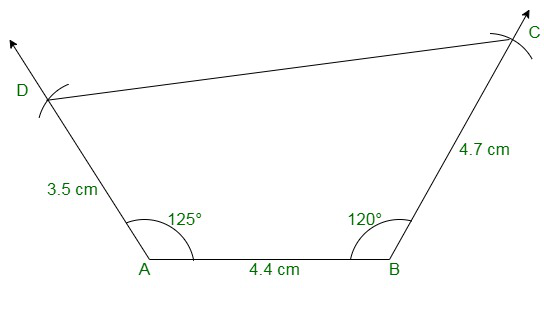### Question 6: Construct a quadrilateral PQRS in which ∠Q = 45° and ∠R = 90°, QR = 5 cm, PQ = 9 cm and RS = 7 cm?

Solution:

We have, ∠Q = 45° and ∠R = 90°, QR = 5 cm, PQ = 9 cm and RS = 7 cm.

Step 1: Draw a line segment QR = 5cm

Step 2: Construct an angle of 45° at Q.

Step 3: Taking Q as the centre, draw an arc radius of 9 cm. Mark this point as P.

Step 4: Construct an angle of 90° at R.

Step 5: Taking R as the centre, draw an arc radius of 7 cm. Mark this point as S.

Step 6: Now join the line segments QP, RS.

Since the line segment, QP and RS are not intersecting at each other, a quadrilateral cannot be formed.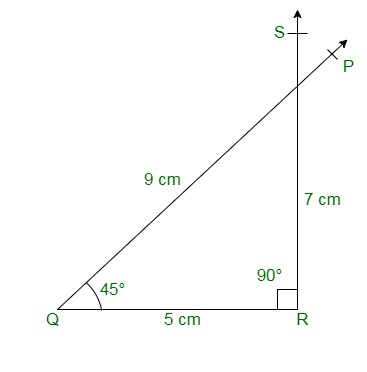### Question 7: Construct a quadrilateral ABCD in which AB = BC = 3 cm, AD = 5 cm, ∠A = 90° and ∠B = 105°?

Solution:

We have, AB = BC = 3 cm, AD = 5 cm, ∠A = 90° and ∠B = 105°.

Step 1: Draw a line AB = 3cm

Step 2: Construct an angle of 90° at A.

Step 3: Cut an arc of radius 5cm with A as the centre to mark that point as D.

Step 4: Construct an angle of 105° at B.

Step 5: Cut an arc of radius 3cm with B as the centre to mark that point as C.

Step 6: Now join AD, BC, and CD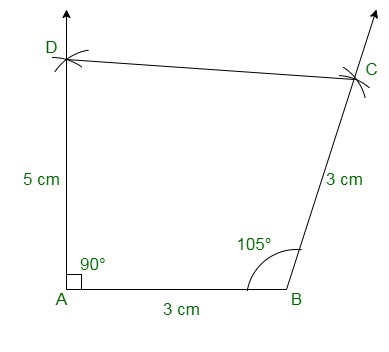### Question 8: Construct a quadrilateral BDEF, where DE = 4.5 cm, EF = 3.5 cm, FB = 6.5 cm, ∠F = 50° and ∠E = 100°?

Solution:

We have, DE = 4.5 cm, EF = 3.5 cm, FB = 6.5 cm, ∠F = 50° and ∠E = 100°.

Step 1: Draw a line EF = 3.5cm

Step 2: Construct an angle of 100° at E.

Step 3: Cut an arc of radius 4.5cm with E as the centre to mark that point as D.

Step 4: Construct an angle of 50° at F.

Step 5: Cut an arc of radius 6.5cm with F as the centre to mark that point as B.

Step 6: Now join DE, FB, and DB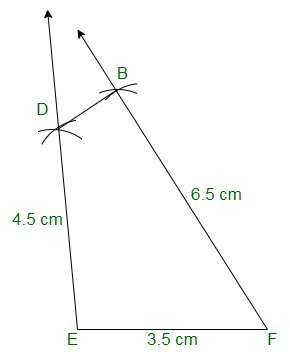My Personal Notes arrow_drop_up